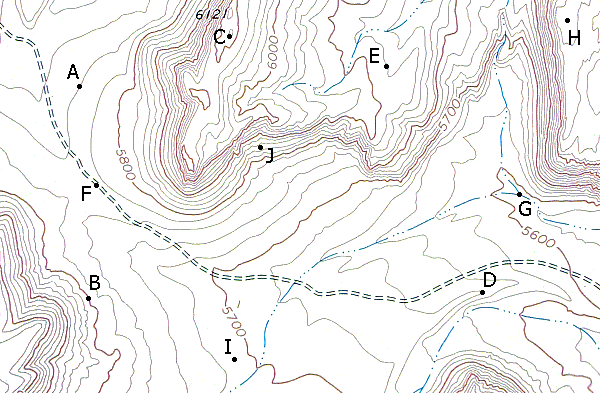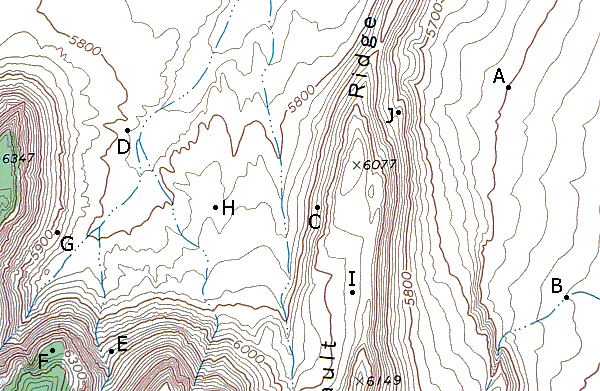# EARTH SCIENCE LAB Topographic Maps

Directions for entering values (unless otherwise directed):
1. Enter all values without commas - example 5,678 would be entered as 5678.
2. Enter all decimal values less than one with a zero before the decimal - example 0.53, 0.32.
3. Round and report all valuess to 2 decimal places. (for example, 10 would have to entered at 10.00)

### Ratio Scales

Convert the ratio scales to verbal scales.

1:10000 = One inch to feet
1:50000 = One inch to feet
1:63360 = One inch to miles
1:100000 = One centemeter to meters
1:100000 = One inch to miles
1:250000 = One centemeter to km
1:250000 = One inch to miles
1:500000 = One centemeter to km
1:500000 = One inch to miles
1:1000000 = One centemeter to km

### Topographic Measurements - Part I

Answer the following questions using the map below.
- Estimate all elevations to the nearest 5 feet.What is the contour interval for this map? feet
What is the elevation of Point A? feet
What is the elevation of Point B? feet
What is the elevation of Point C? feet
What is the elevation of Point D? feet
What is the elevation of Point E? feet
What is the elevation of Point F? feet
What is the elevation of Point G? feet
What is the elevation of Point H? feet
What is the elevation of Point I? feet
What is the elevation of Point J? feet
What is the relief between Points C and F? feet

### Topographic Measurements - Part II

Answer the following questions using the map below.
- Estimate all elevations to the nearest 5 feet.